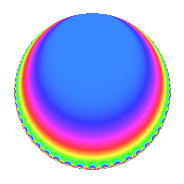Properties

 Label 3600.1.e.bLevel 3600 Weight 1 Character orbit 3600.e Self dual Yes Analytic conductor 1.797 Analytic rank 0 Dimension 1 Projective image $$D_{2}$$ CM/RM disc. -3, -4, 12 Inner twists 4

Related objects

Newspace parameters

 Level: $$N$$ = $$3600 = 2^{4} \cdot 3^{2} \cdot 5^{2}$$ Weight: $$k$$ = $$1$$ Character orbit: $$[\chi]$$ = 3600.e (of order $$2$$ and degree $$1$$)

Newform invariants

 Self dual: Yes Analytic conductor: $$1.79663404548$$ Analytic rank: $$0$$ Dimension: $$1$$ Coefficient field: $$\mathbb{Q}$$ Coefficient ring: $$\mathbb{Z}$$ Coefficient ring index: $$1$$ Projective image $$D_{2}$$ Projective field Galois closure of $$\Q(\zeta_{12})$$ Artin image size $$8$$ Artin image $D_4$ Artin field Galois closure of 4.0.10800.2

$q$-expansion

 $$f(q)$$ $$=$$ $$q$$ $$\mathstrut +\mathstrut O(q^{10})$$ $$q$$ $$\mathstrut +\mathstrut 2q^{13}$$ $$\mathstrut -\mathstrut 2q^{37}$$ $$\mathstrut +\mathstrut q^{49}$$ $$\mathstrut +\mathstrut 2q^{61}$$ $$\mathstrut +\mathstrut 2q^{73}$$ $$\mathstrut +\mathstrut 2q^{97}$$ $$\mathstrut +\mathstrut O(q^{100})$$

Character Values

We give the values of $$\chi$$ on generators for $$\left(\mathbb{Z}/3600\mathbb{Z}\right)^\times$$.

 $$n$$ $$577$$ $$901$$ $$2801$$ $$3151$$ $$\chi(n)$$ $$1$$ $$1$$ $$1$$ $$-1$$

Embeddings

For each embedding $$\iota_m$$ of the coefficient field, the values $$\iota_m(a_n)$$ are shown below.

For more information on an embedded modular form you can click on its label.

Label $$\iota_m(\nu)$$ $$a_{2}$$ $$a_{3}$$ $$a_{4}$$ $$a_{5}$$ $$a_{6}$$ $$a_{7}$$ $$a_{8}$$ $$a_{9}$$ $$a_{10}$$
3151.1
 0
0 0 0 0 0 0 0 0 0
 $$n$$: e.g. 2-40 or 990-1000 Significant digits: Format: Complex embeddings Normalized embeddings Satake parameters Satake angles

Inner twists

Char. orbit Parity Mult. Self Twist Proved
1.a Even 1 trivial yes
3.b Odd 1 CM by $$\Q(\sqrt{-3})$$ yes
4.b Odd 1 CM by $$\Q(\sqrt{-1})$$ yes
12.b Even 1 RM by $$\Q(\sqrt{3})$$ yes

Hecke kernels

This newform can be constructed as the intersection of the kernels of the following linear operators acting on $$S_{1}^{\mathrm{new}}(3600, [\chi])$$:

 $$T_{7}$$ $$T_{13}$$ $$\mathstrut -\mathstrut 2$$